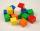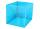# Right triangle + cube - math problems

#### Number of problems found: 30

• CubeCalculate the surface of the cube ABCDA'B'C'D' if the area of rectangle ACC'A' = 344 mm2.
• Body diagonalFind the cube surface if its body diagonal has a size of 6 cm.
• Cube - wallV kocke ABCDEFGH je |CF|=4 √ 2. Aký je povrch kocky?
• Center of the cubeThe Center of the cube has a distance 16 cm from each vertex. Calculate the volume V and surface area S of the cube.
• Rotary coneThe volume of the rotation of the cone is 472 cm3, and the angle between the side of the cone and the base angle is 70°. Calculate the lateral surface area of this cone.I have homework. The cube has an edge 7 cm long and I must find wall and body diagonal.
• PrismRight-angled prism, whose base is a right triangle with leg a = 3 cm and hypotenuse c = 13 cm, has the same volume as a cube with an edge length of 3 dm. a) Find the height of the prism b) Calculate the surface of the prism c) What percentage of the cube'
• CubesOne cube is an inscribed sphere and the other one described. Calculate the difference of volumes of cubes, if the difference of surfaces in 257 mm2.
• Cube - anglesCalculate the angle between the wall diagonal and cube base. Calculate the angle between the cube body diagonal and cube base.
• Cube diagonalDetermine the length of the cube diagonal with edge 37 mm.

We apologize, but in this category are not a lot of examples.
Do you have an exciting math question or word problem that you can't solve? Ask a question or post a math problem, and we can try to solve it.

We will send a solution to your e-mail address. Solved examples are also published here. Please enter the e-mail correctly and check whether you don't have a full mailbox.